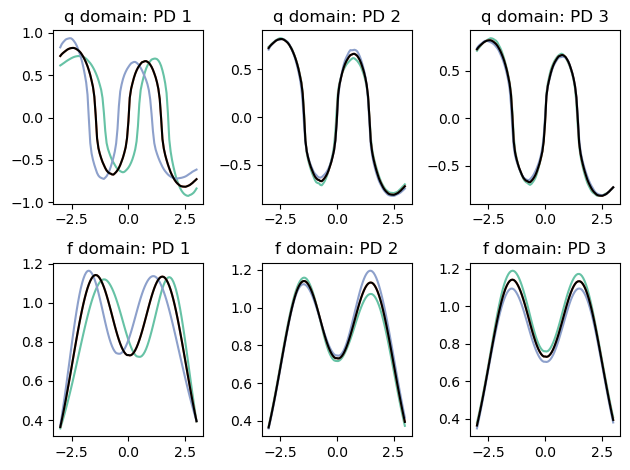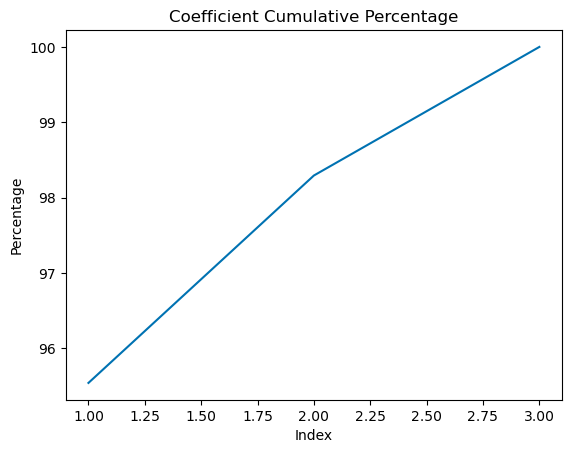# Elastic Functional Principal Component Analysis¶

After we have aligned our data we can compute functional principal component analysis (fPCA) on the aligned data, warping functions, and jointly

:

import fdasrsf as fs
import numpy as np


We will load in our example data again and compute the alignment

:

data = np.load('../../bin/simu_data.npz')
time = data['arr_1']
f = data['arr_0']
obj = fs.fdawarp(f,time)
obj.srsf_align(parallel=True)

Initializing...
Compute Karcher Mean of 21 function in SRSF space...
updating step: r=1
updating step: r=2


## Vertical fPCA¶

We will first compute fPCA on the aligned functions, by constructing the object and computing the PCA for the number of components, default=3)

:

vpca = fs.fdavpca(obj)
vpca.calc_fpca(no=3)


We then can plot the principal directions

:

vpca.plot()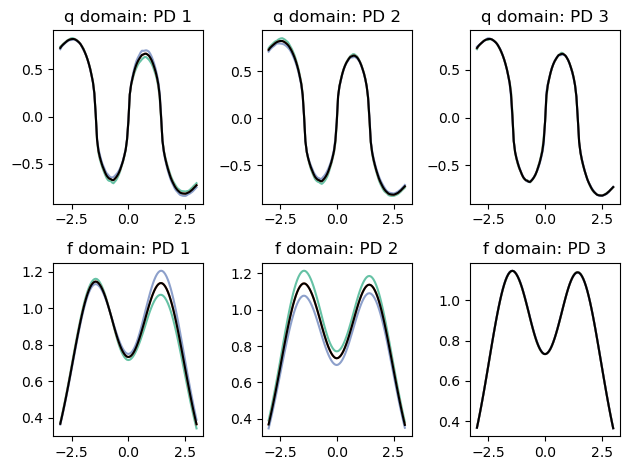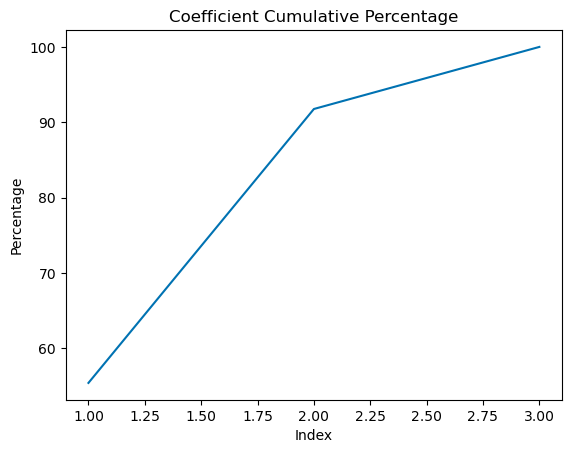## Horizontal fPCA¶

We can then compute PCA on the set of warping functions

:

hpca = fs.fdahpca(obj)
hpca.calc_fpca(no=3)


We then can plot the principal directions

:

hpca.plot()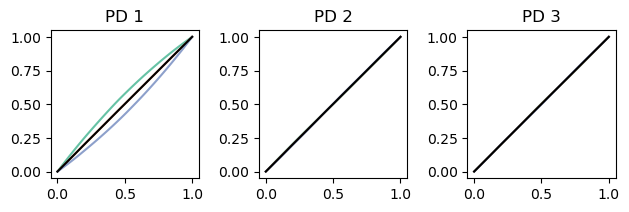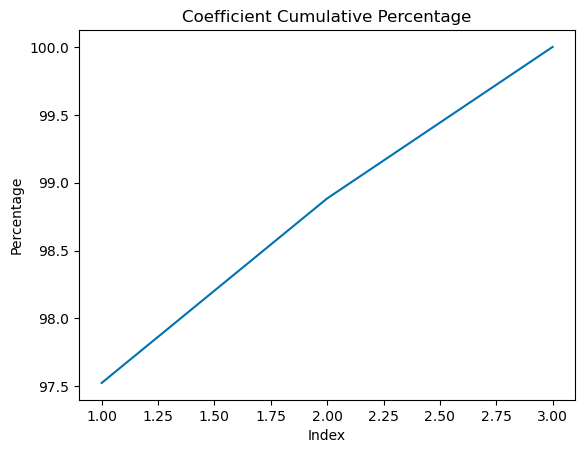## Joint fPCA¶

We can also compute the fPCA on jointly on the phase/amplitude space if we feel there is correlation between the variabilities

:

jpca = fs.fdajpca(obj)
jpca.calc_fpca(no=3)


We then can plot the principal directions

:

jpca.plot()